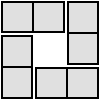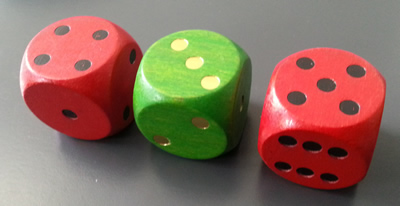#### You may also like### Consecutive Numbers

An investigation involving adding and subtracting sets of consecutive numbers. Lots to find out, lots to explore.### Domino Square

Use the 'double-3 down' dominoes to make a square so that each side has eight dots.### 4 Dom

Use these four dominoes to make a square that has the same number of dots on each side.

# Roll These Dice

##### Age 7 to 11Challenge LevelTwo dice are RED and one is GREEN.

Roll the dice and add up the numbers on the two RED dice and then subtract the number on the GREEN.

So if one RED is $4$ and the other RED is $5$ and the GREEN is $3$ we should add together $4$ and $5$ to make $9$ and then subtract the $3$ so that gives us a final answer of $6$.

You'll need to roll these dice many times and see what numbers you make each time by doing the addition and subtraction.

In this game it would be good to find out:-

• what are the final answers by doing the addition and subtraction each time?
• what are all the different possible numbers?
• is there a good way of making sure you find them all?
• how will you record what you've found out?

Now have a go!

Look at your results and write down some questions that you could ask about them. For example, do any of them have the same answers? If so, why?

Then you could ask yourself, "I wonder what would happen if, instead, I ...?''

Printable NRICH Roadshow resource.